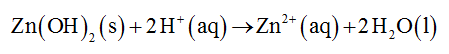# Zn(II) hydroxide is amphoteric (amphiprotic). Complete and balance the following equations.Include the phases of all species in the chemical equations.Complete and balance a chemical equation showing how an aqueous suspension of this compound reacts to the addition of a strong acid.Zn(OH)2​(s)+2H+(aq) Complete and balance a chemical equation showing how an aqueous suspension of this compound reacts to the addition of a strong base. Zn(OH)2​(s)+2OH−(aq)

Question
98 views

Zn(II) hydroxide is amphoteric (amphiprotic). Complete and balance the following equations.

Include the phases of all species in the chemical equations.
Complete and balance a chemical equation showing how an aqueous suspension of this compound reacts to the addition of a strong acid.

Zn(OH)2​(s)+2H+(aq)

Complete and balance a chemical equation showing how an aqueous suspension of this compound reacts to the addition of a strong base.

Zn(OH)2​(s)+2OH−(aq)
check_circle

Step 1

Balanced chemical equation showing how an aqueous suspension of this compound reacts to the addition of a strong acid is given below....

### Want to see the full answer?

See Solution

#### Want to see this answer and more?

Solutions are written by subject experts who are available 24/7. Questions are typically answered within 1 hour.*

See Solution
*Response times may vary by subject and question.
Tagged in